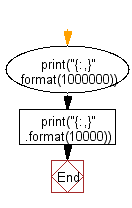﻿ Python Math: Print number with commas as thousands separators (from right side) - w3resource# Python Math: Print number with commas as thousands separators

## Python Math: Exercise-26 with Solution

Write a Python program to print number with commas as thousands separators (from right side).

Sample Solution:-

Python Code:

``````print("{:,}".format(1000000))
print("{:,}".format(10000))
```
```

Sample Output:

```1,000,000
10,000
```

Pictorial Presentation:Flowchart:Python Code Editor:

Have another way to solve this solution? Contribute your code (and comments) through Disqus.

What is the difficulty level of this exercise?

Test your Programming skills with w3resource's quiz.

﻿

```>>> students = [{'name': 'John', 'score': 98}, {'name': 'Mike', 'score': 94}, {'name': 'Jennifer', 'score': 99}]